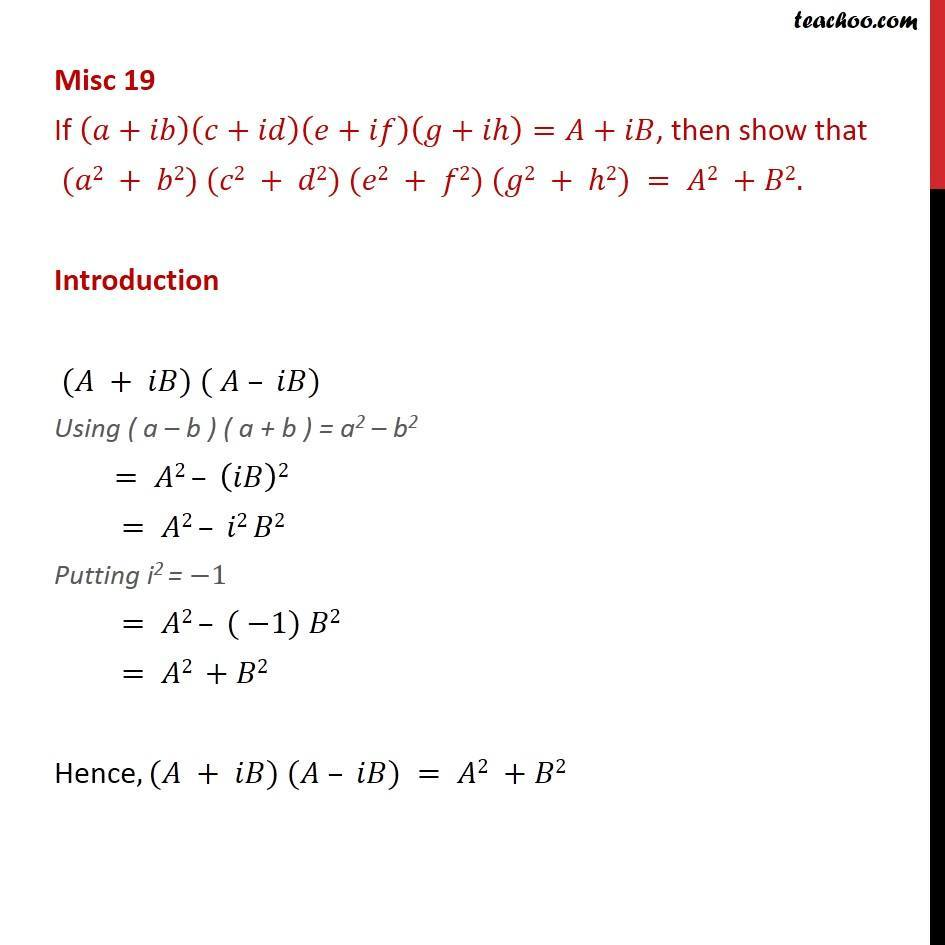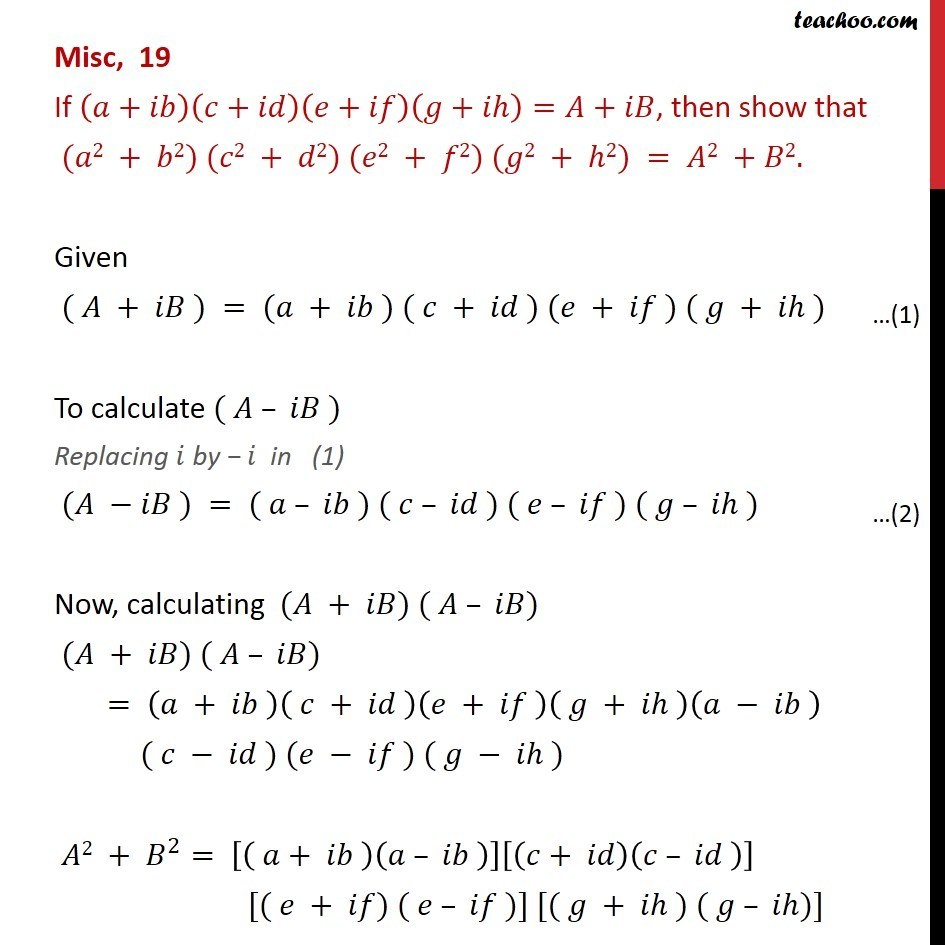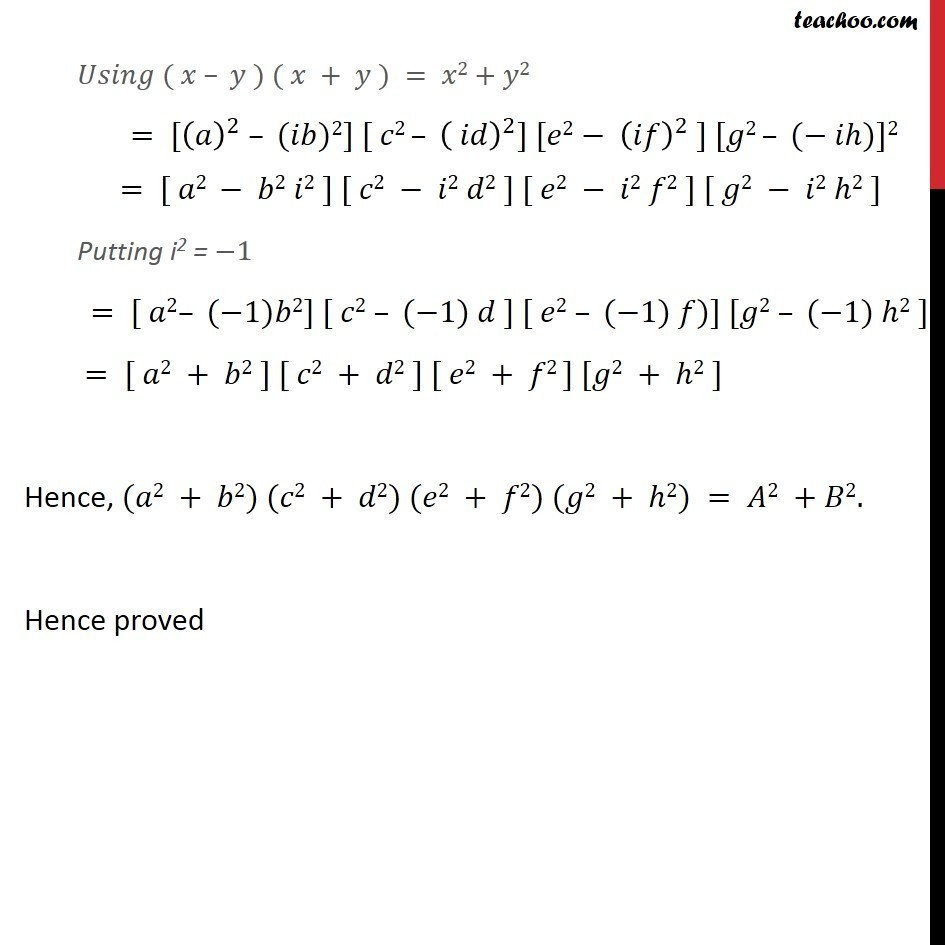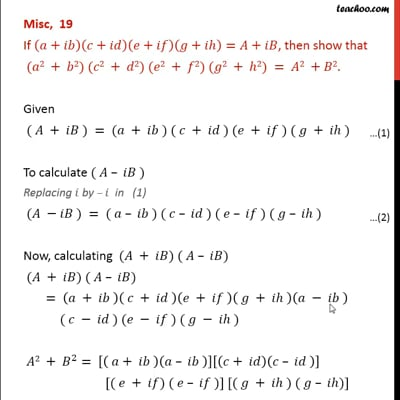Miscellaneous

Chapter 5 Class 11 Complex Numbers
Serial order wiseThis video is only available for Teachoo black users

Solve all your doubts with Teachoo Black (new monthly pack available now!)

### Transcript

Misc 19 If (𝑎+𝑖𝑏)(𝑐+𝑖𝑑)(𝑒+𝑖𝑓)(𝑔+𝑖ℎ)=𝐴+𝑖𝐵, then show that (𝑎2 + 𝑏2) (𝑐2 + 𝑑2) (𝑒2 + 𝑓2) (𝑔2 + ℎ2) = 𝐴2 +𝐵2. Introduction (𝐴 + 𝑖𝐵) ( 𝐴 – 𝑖𝐵) Using ( a – b ) ( a + b ) = a2 – b2 = 𝐴2 – (𝑖𝐵)2 = 𝐴2 – 𝑖2 𝐵2 Putting i2 = −1 = 𝐴2 – ( −1) 𝐵2 = 𝐴2 +𝐵2 Hence, (𝐴 + 𝑖𝐵) (𝐴 – 𝑖𝐵) = 𝐴2 +𝐵2 Misc, 19 If (𝑎+𝑖𝑏)(𝑐+𝑖𝑑)(𝑒+𝑖𝑓)(𝑔+𝑖ℎ)=𝐴+𝑖𝐵, then show that (𝑎2 + 𝑏2) (𝑐2 + 𝑑2) (𝑒2 + 𝑓2) (𝑔2 + ℎ2) = 𝐴2 +𝐵2. Given ( 𝐴 + 𝑖𝐵 ) = (𝑎 + 𝑖𝑏 ) ( 𝑐 + 𝑖𝑑 ) (𝑒 + 𝑖𝑓 ) ( 𝑔 + 𝑖ℎ ) To calculate ( 𝐴 – 𝑖𝐵 ) Replacing 𝑖 by –𝑖 in (1) (𝐴 −𝑖𝐵 ) = ( 𝑎 – 𝑖𝑏 ) ( 𝑐 – 𝑖𝑑 ) ( 𝑒 – 𝑖𝑓 ) ( 𝑔 – 𝑖ℎ ) Now, calculating (𝐴 + 𝑖𝐵) ( 𝐴 – 𝑖𝐵) (𝐴 + 𝑖𝐵) ( 𝐴 – 𝑖𝐵) = (𝑎 + 𝑖𝑏 )( 𝑐 + 𝑖𝑑 )(𝑒 + 𝑖𝑓 )( 𝑔 + 𝑖ℎ )(𝑎 − 𝑖𝑏 ) ( 𝑐 − 𝑖𝑑 ) (𝑒 − 𝑖𝑓 ) ( 𝑔 − 𝑖ℎ ) 𝐴2 + 𝐵^2= [( 𝑎+ 𝑖𝑏 )(𝑎 – 𝑖𝑏 )][(𝑐+ 𝑖𝑑)(𝑐 – 𝑖𝑑 )] [( 𝑒 + 𝑖𝑓) ( 𝑒 – 𝑖𝑓 )] [( 𝑔 + 𝑖ℎ ) ( 𝑔 – 𝑖ℎ)] 𝑈𝑠𝑖𝑛𝑔 ( 𝑥 – 𝑦 ) ( 𝑥 + 𝑦 ) = 𝑥2+𝑦2 = [(𝑎)^2 – (𝑖𝑏)2] [ 𝑐2 – ( 𝑖𝑑)^2] [𝑒2− (𝑖𝑓)^2 ] [𝑔2 – (− 𝑖ℎ)]2 = [ 𝑎2 − 𝑏2 𝑖2 ] [ 𝑐2 − 𝑖2 𝑑2 ] [ 𝑒2 − 𝑖2 𝑓2 ] [ 𝑔2 − 𝑖2 ℎ2 ] Putting i2 = −1 = [ 𝑎2– (−1)𝑏2] [ 𝑐2 – (−1) 𝑑 ] [ 𝑒2 – (−1) 𝑓)] [𝑔2 – (−1) ℎ2 ] = [ 𝑎2 + 𝑏2 ] [ 𝑐2 + 𝑑2 ] [ 𝑒2 + 𝑓2 ] [𝑔2 + ℎ2 ] Hence, (𝑎2 + 𝑏2) (𝑐2 + 𝑑2) (𝑒2 + 𝑓2) (𝑔2 + ℎ2) = 𝐴2 +𝐵2. Hence proved